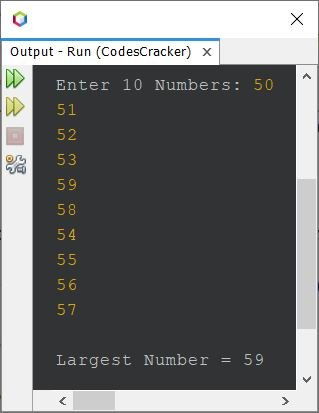# Java Program to Find Largest Number in an Array

## Java Find Largest Number in Array using for Loop

The question is, write a Java program to find and print the largest number in an array of 10 numbers. The program given below is its answer:

```import java.util.Scanner;

public class CodesCracker
{
public static void main(String[] args)
{
int i, large;
int[] arr = new int;
Scanner scan = new Scanner(System.in);

System.out.print("Enter 10 Numbers: ");
for(i=0; i<10; i++)
arr[i] = scan.nextInt();

large = arr;
for(i=1; i<10; i++)
{
if(large<arr[i])
large = arr[i];
}

System.out.println("\nLargest Number = " +large);
}
}```

The snapshot given below shows the sample run of above program, with user input 50, 51, 52, 53, 59, 58, 54, 55, 56, 57 as ten numbers for the array to find and print the largest one, among all these ten numbers:The above program only works with 10 numbers. Therefore let's modify the program and create another, that find and prints largest number in an array of n numbers:

```import java.util.Scanner;

public class CodesCracker
{
public static void main(String[] args)
{
int n, i, large;
Scanner scan = new Scanner(System.in);

System.out.print("Enter the Value of n: ");
n = scan.nextInt();
int[] arr = new int[n];
System.out.print("Enter " +n+ " Numbers: ");
for(i=0; i<n; i++)
arr[i] = scan.nextInt();

large = arr;
for(i=1; i<n; i++)
{
if(large<arr[i])
large = arr[i];
}

System.out.println("\nLargest Number = " +large);
}
}```

The sample run with user input 6 as size or value of n and 1, 2, 3, 4, 5, 6 as six elements or numbers is shown in the snapshot given below:## Java Find Largest Number in Array using while Loop

```import java.util.Scanner;

public class CodesCracker
{
public static void main(String[] args)
{
Scanner scan = new Scanner(System.in);

System.out.print("Enter the Value of n: ");
int n = scan.nextInt();
int[] arr = new int[n];
System.out.print("Enter " +n+ " Numbers: ");
int i=0;
while(i<n)
{
arr[i] = scan.nextInt();
i++;
}

int large = arr;
i = 1;
while(i<n)
{
if(large<arr[i])
large = arr[i];
i++;
}

System.out.println("\nLargest Number = " +large);
}
}```

This program produces same output as of previous program.

#### Same Program in Other Languages

Java Online Test

« Previous Program Next Program »